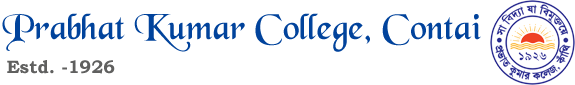CRITERIA FOR ADMISSION (UG), SESSION -2019-20

A) HONOURS COURSE :

 B.Com (Hons.) Total – 250 and Accountancy – 50 and Madhyamik Math.– 45 or BEBM/ STAT- 45. For Science Students Total – 300 and Passed in H.S. Math. B.Sc (Hons.) in Anthropology Total – 250 and Anthropology (Th.) – 35 or Biology (Th.)–35, B.A. (Hons.) in Bengali Total – 300 and Bengali – 60, B.Sc (Hons.) in Botany Total – 300 and Biology (Th.) – 42, B.Sc (Hons.) in Chemistry Total – 300 and Chemistry (Th.) – 42, Math. – 40, B.Sc (Hons.) in Computer Science Total – 250 and passed in Math (H.S.), B.Sc (Hons.) in Economics Total – 250 and Math.–30, or Madhyamik Math.– 45, B.A. (Hons.) in Education Total – 250 and Education – 60, B.A. (Hons.) in English Total – 300 and English – 60, B.Sc (Hons.) in Geography Total – 300 and Geography (Th.) – 42, B.A. (Hons.) in History Total – 225 and History – 50, B.Sc (Hons.) in Mathematics Total – 300 and Mathematics – 60, B.A. (Hons.) in Music Total – 250 or Music (Th.) – 25 B.Sc (Hons.) in Nutrition Total – 300 and Nutrition or Biology (Th.)- 50  and Chem. (Th.)- 35, B.A. (Hons.) in Philosophy Total – 225 and Philosophy – 50, B.Sc (Hons.) in Physics Total – 300 and Physics (Th.) – 42, Math. – 50, B.A. (Hons.) in Pol. Science Total – 225 and Pol. Science – 50, B.A. (Hons.) in Sanskrit Total – 250 and Sanskrit – 50, B.A. (Hons.) in Sociology Total – 225 and Sociology– 50, B.Sc (Hons.) in Zoology Total – 300 and Biology (Th.) – 42, Aquaculture & Management Total – 250 and Biology (Th.) – 35

B) GENERAL COURSE :

 SUBJECT FOR ALL CANDIDATES B.A. Passed in H.S. with 40% marks secured in best five subjects B.Com. Passed in H.S. with 40% marks secured in best five subjects B.Sc. Passed in H.S. with 45% marks secured in best five SCIENCE subjects B.C.A. Total – 225 & 40% in Math. (H.S.) N.B.: For reserve students’ marks relaxation & seat reservation as per Govt. rules.

July2

June24